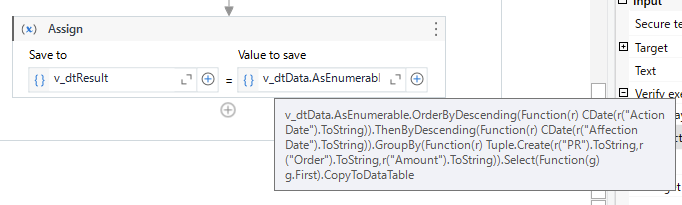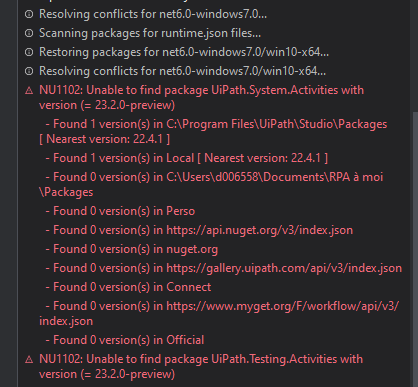# Problem with Linq query with Group By

Final Edit: In fact the code given by ChatGPT works fine, the problem was further down the loop which processed the v_dtResult datatable and looped over the first row of the table. The real problem was therefore between the chair and the keyboardso you can take the code from ChatGPT or from Yoichi, both work

Regards, Olivier

Hi All

I tried to do a linq query by myself but it didn’t work so I asked chatGPT and it gave me something, but the result is not good. Here is the problem:

I have a datatable v_dtData as below (it’s a sample)

I want to group by PR, Order and Amount, then, order by Action Date Descending then by Affection Date descending and copy the first row of the group by in a new datatable

here is the code from ChatGPT:

v_dtResult = v_dtData.AsEnumerable() _
.GroupBy(Function(row) New With { _
Key .PR = row(“PR”).ToString, _
Key .Commande = row(“Order”).ToString,
Key .Montant = convert.ToDouble(row(“Amount”))
}) _
.Select(Function(Group) Group.First()) _
.OrderByDescending(Function(row) row.Field(Of DateTime)(“action date”)) _
.ThenByDescending(Function(row) row.Field(Of DateTime)(“affectation date”)) _
.CopyToDataTable()

the problem is that every row of the v_dtResult are identical, the query only the first group by is processed and repeated until the end. ChatGPT tells me something about class Anonymous and ask me to change for:

Public Class GroupKey
Public Property PR As String
Public Property Commande As String
Public Property Montant As Double
End Class

v_dtResul = v_dtData.AsEnumerable() _
.OrderByDescending(Function(row) row.Field(Of DateTime)(“action date”)) _
.ThenByDescending(Function(row) row.Field(Of DateTime)(“affectation date”)) _
.GroupBy(Function(row) New GroupKey With { _
.PR = row(“PR”).ToString(), _
.Commande = row(“order”).ToString(), _
.Montant = Convert.ToDouble(row(“amount”)) _
}) _
.Select(Function(Group) Group.First()) _
.CopyToDataTable()

but I can’t manage how to implement that in an assign

Can somebody help me ?

1 Like

@olivier.garcin

Welcome to the Community

`dt.AsEnumerable.GroupBy(function(x) {x("PR").ToString,x("Order and Amount").ToString}).Select(function(x) x.OrderByDescending(function(y) Cdate(y("Action Date").ToString)).OrderByDescending(function(y) Cdate(y("Affection Date").ToString)).First).CopyToDataTable`

Hope this helps

Cheers

Hi Anil, thanks for your help

I tried your code with adaptation to real name columns name but the compilation failed
I dont’ know if you notice but Amount (Montant in French) is Double, not String
here is the code I tried to run:

v_dtData.AsEnumerable() _
.GroupBy(Function(x) {
x(“PR”).toString,
x(“Commande”).tostring,
x(“Montant”).ToString
}) _
.Select(Function(x) x.OrderByDescending(Function(y) CDate(y(“Date Action”).ToString)).OrderByDescending(Function(y) CDate(y(“Date Affectation”).ToString)).First).CopyToDataTable

Hi,

Can you try either of the following?

``````v_dtResult = v_dtData.AsEnumerable.OrderByDescending(Function(r) DateTime.ParseExact(r("Action Date").ToString,"dd/MM/yyyy HH:mm",System.Globalization.CultureInfo.InvariantCulture)).ThenByDescending(Function(r) DateTime.ParseExact(r("Affection Date").ToString,"dd/MM/yyyy",System.Globalization.CultureInfo.InvariantCulture)).GroupBy(Function(r) Tuple.Create(r("PR").ToString,r("Order").ToString,r("Amount").ToString)).Select(Function(g) g.First).CopyToDataTable
``````

OR

``````v_dtResult = v_dtData.AsEnumerable.OrderByDescending(Function(r) CDate(r("Action Date").ToString)).ThenByDescending(Function(r) CDate(r("Affection Date").ToString)).GroupBy(Function(r) Tuple.Create(r("PR").ToString,r("Order").ToString,r("Amount").ToString)).Select(Function(g) g.First).CopyToDataTable
``````

note: It depends on type (text or date) of date data in worksheet.

Regards,

1 Like

@olivier.garcin

Can you show what error you got in compilation?

Cheers

Hi Yoichi, thanks for your help

I have the same problem with both of your solution, I have a lot of error during compilation. Here is the beginnig of the error compilation message:

An unexpected error occurred during the library compilation process:
Compiling the assembly returned the following errors:

• Expected ‘End Function’.
• Expression expected.
• This statement cannot appear in the method body. It is interpreted as the end of the method

Hi,

Can you try this in Assign activity as the following?Regards,

it’s already what I am doing:
Maybe there is a problem with my version of .NET ?

Hi,

Can you share your xaml file?

Regards,

@olivier.garcin

Can you confirm if your is c# or vb.net ?

Also can you share a screenshot of how you are using that might help better…if you can include the exact exception message it could help Or your xaml… because the query given to you is a compiled one in vb.net prject type

Cheers

I’m a new member so I can’t right now upload file … need to go in 5 topics, read 30 posts. I come backHere are my files:

test_Linq.xlsx (12.6 KB)
test_linq.xaml (8.1 KB)

thanks

Hi,

In my environment, the following expression works.

``````v_dtDataF =  v_dtData.AsEnumerable.OrderByDescending(Function(r) CDate(r("Action Date").ToString)).ThenByDescending(Function(r) CDate(r("Affectation Date").ToString)).GroupBy(Function(r) Tuple.Create(r("PR").ToString,r("Order").ToString,r("Amount").ToString)).Select(Function(g) g.First).CopyToDataTable
``````

Can you try the following sample?

Sample20230318-2.zip (13.0 KB)

Regards,

Unfortunately, I can’t open your Main because my version is 2022.4 and your Main need something more recentI copy my test_ling to a brand new Main file and I don’t have any compilation error, and it’s works
I think my original file is broken … My problem seems to be gone, I have to check with a bigger sample

I will let you know2 Likes

It works, I selected the last code from Yoichi as Solution, please change that if it’s not correct from your point of vue

Thanks A LOT for your helps Guys, you’re smarter than ChatGPTThis topic was automatically closed 3 days after the last reply. New replies are no longer allowed.